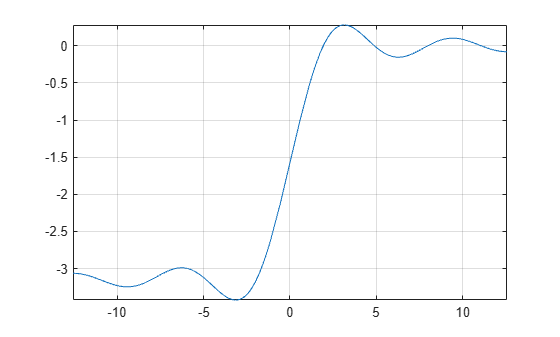# ssinint

Shifted sine integral function

## Syntax

``ssinint(X)``

## Description

example

````ssinint(X)` returns the shifted sine integral function `ssinint(X) = sinint(X) — pi/2`. ```

## Examples

### Shifted Sine Integral Function for Numeric and Symbolic Arguments

Depending on its arguments, `ssinint` returns floating-point or exact symbolic results.

Compute the shifted sine integral function for these numbers. Because these numbers are not symbolic objects, `ssinint` returns floating-point results.

`A = ssinint([- pi, 0, pi/2, pi, 1])`
```A = -3.4227 -1.5708 -0.2000 0.2811 -0.6247```

Compute the shifted sine integral function for the numbers converted to symbolic objects. For many symbolic (exact) numbers, `ssinint` returns unresolved symbolic calls.

`symA = ssinint(sym([- pi, 0, pi/2, pi, 1]))`
```symA = [ - pi - ssinint(pi), -pi/2, ssinint(pi/2), ssinint(pi), ssinint(1)]```

Use `vpa` to approximate symbolic results with floating-point numbers:

`vpa(symA)`
```ans = [ -3.4227333787773627895923750617977,... -1.5707963267948966192313216916398,... -0.20003415864040813916164340325818,... 0.28114072518756955112973167851824,... -0.62471325642771360428996837781657]```

### Plot Shifted Sine Integral Function

Plot the shifted sine integral function on the interval from `-4*pi` to `4*pi`.

```syms x fplot(ssinint(x),[-4*pi 4*pi]) grid on```### Handle Expressions Containing Shifted Sine Integral Function

Many functions, such as `diff`, `int`, and `taylor`, can handle expressions containing `ssinint`.

Find the first and second derivatives of the shifted sine integral function:

```syms x diff(ssinint(x), x) diff(ssinint(x), x, x)```
```ans = sin(x)/x ans = cos(x)/x - sin(x)/x^2```

Find the indefinite integral of the shifted sine integral function:

`int(ssinint(x), x)`
```ans = cos(x) + x*ssinint(x)```

Find the Taylor series expansion of `ssinint(x)`:

`taylor(ssinint(x), x)`
```ans = x^5/600 - x^3/18 + x - pi/2```

## Input Arguments

collapse all

Input, specified as a symbolic number, variable, expression, or function, or as a vector or matrix of symbolic numbers, variables, expressions, or functions.

collapse all

### Sine Integral Function

The sine integral function is defined as follows:

`$\text{Si}\left(x\right)=\underset{0}{\overset{x}{\int }}\frac{\mathrm{sin}\left(t\right)}{t}dt$`

### Shifted Sine Integral Function

The sine integral function is defined as Ssi(x) = Si(x) - π/2.

 Gautschi, W. and W. F. Cahill. “Exponential Integral and Related Functions.” Handbook of Mathematical Functions with Formulas, Graphs, and Mathematical Tables. (M. Abramowitz and I. A. Stegun, eds.). New York: Dover, 1972.